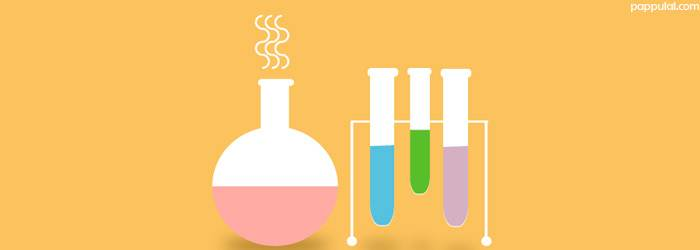Medical Sample papers

JEE questions on Mole ConceptMole Concept IIT JEE questions with answers:

Ques. Which one of the following pairs of gases contains the same number of molecules
(a) 16 g of O2 and 14 g of N2
(b) 8 g of O2 and 22 g of CO2
(c) 28 g of N2 and 22 g of CO2
(d) 32 g of O2 and 32 g of N2
Ans. (a)

Ques. Number of gm of oxygen in 32.2 g Na2SO4 . 10H2O is
(a) 20.8
(b) 22.4
(c) 2.24
(d) 2.08
Ans. (b)

Ques. 250 ml of a sodium carbonate solution contains 2.65 grams of Na2CO3. If 10 ml of this solution is diluted to one litre, what is the concentration of the resultant solution (mol. wt. of Na2CO3 = 106)
(a) 0.1 M
(b) 0.001 M
(c) 0.01 M
(d) 10–4
Ans. (b)

Ques. A molar solution is one that contains one mole of a solute in
(a) 1000 g of the solvent
(b) One litre of the solvent
(c) One litre of the solution
(d) 22.4 litres of the solution
Ans. (c)

Ques. The number of water molecules in 1 litre of water is
(a) 18
(b) 18 x 1000
(c) NA
(d) 55.55 NA
Ans. (d)

Related: Fungi test questions

Ques. The numbers of moles of BaCO3 which contain 1.5 moles of oxygen atoms is
(a) 0.5
(b) 1
(c) 3
(d) 6.02 x 1023
Ans. (a)

Ques. Number of molecules in 100 ml of each of O2, NH3 and CO2 at STP are
(a) In the order CO2 < O2 < NH3
(b) In the order NH3 < O2 < CO2
(c) The same
(d) NH3 = CO2 < O2
Ans. (c)

Ques. The total number of protons in 10 g of calcium carbonate is (N0 = 6.023 x 1023)
(a) 1.5057 x 1024
(b) 2.0478 x 1024
(c) 3.0115 x 1024
(d) 4.0956 x 1024
Ans. (c)

Ques. The total number of gm-molecules of SO2Cl2 in 13.5 g of sulphuryl chloride is
(a) 0.1
(b) 0.2
(c) 0.3
(d) 0.4
Ans. (a)

Ques. The largest number of molecules is in
(a) 34 g of water
(b) 28 g of CO2
(c) 46 g of CH3OH
(d) 54 g of N2O5
Ans. (a)

Ques. 2g of oxygen contains number of atoms equal to that in
(a) 0.5 g of hydrogen
(b) 4g of sulphur
(c) 7g of nitrogen
(d) 2.3 g of sodium
Ans. (b)

Ques. One mole of calcium phosphide on reaction with excess of water gives
(a) One mole of phosphine
(b) Two moles of phosphoric acid
(c) Two moles of phosphine
(d) One mole of phosphorus pentoxide
Ans. (c)

Ques. Molarity of liquid HCl with density equal to 1.17 g / cc is
(a) 36.5
(b) 18.25
(c) 32.05
(d) 4.65
Ans. (c)